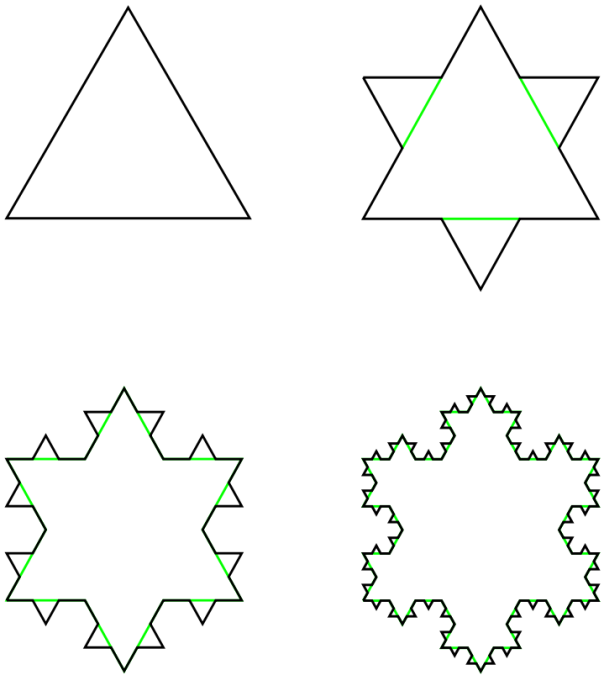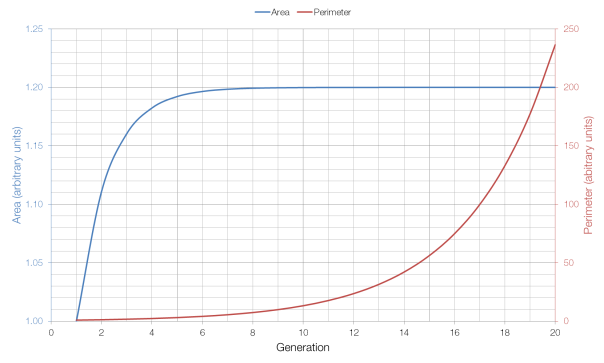# One Million Hats

From the always-excellent Futility Closet, a problem by Paul J. Nahin:

Each of a million people puts his or her hat into a very large box. Each hat has its owner’s name on it. The box is given a good shaking, and then each person, one after another, randomly draws a hat out of the box. What is the probability that at least one person gets their own hat back?

Most people might think that the chance is very, very small, but it’s not. It’s actually more than 60%. How can this be true?

We can view this problem as having only two possible solutions (events): either nobody gets their hat back, or at least one person gets their hat back. The sum of the probabilities of these events must be equal to one and therefore if we can work out the probability that nobody gets their own hat back, then the probability that at least one person does is one minus that.

For each person, there is only a one-in-a-million chance that they have picked their own hat, and thus a 999999 in 1000000 chance that they have not got their own hat.$\Pr \left( \textnormal{one incorrect hat} \right) = \frac{999999}{1000000}$$\Pr \left( \textnormal{one million incorrect hats} \right) = \left( \frac{999999}{1000000} \right)^{1000000}$$\Pr \left( \textnormal{one million incorrect hats} \right) = 0.368$

If the probability of all one million people picking the incorrect hat in 0.368, then via our previous reasoning, the probability of at least one person picking the correct hat is 1-0.368, or 63.2%.

This is a very counterintuitive result, but the wording of the question is key. If we changed the wording to exactly one person getting their hat back then our answer changes dramatically. Starting with our 63.2% chance, we would have to subtract the chance of two people getting their hats back, and of three people getting their hats back, and so on … until we reached the very small chance of one person, and one person only, getting their hat back.

# Fixed Area, Infinite Perimeter

The Koch Snowflake (named after its inventor, the Swedish mathematician Helge von Koch) is a fractal with a number of interesting properties.The first four generations of the Koch Snowflake

As the number of generations increases, the area of the snowflake increases, but it increases towards a limit: eight-fifths of the size of the first (triangular) generation. This is because each additional generation adds three triangles which are one-ninth the size of the triangle added in the previous generation (for a total increase of one-third), and the additional of increasingly small triangles has a lesser and lesser effect on the overall area as the number of generations increases.

But as the number of generations increases, the perimeter of the shape continues to grow, without approaching a limit: each generation of the Snowflake has a perimeter which is four-thirds of the previous generation’s perimeter. This is because the additional perimeter added each time is four times one-third of the length of each side, for a total increase of four-thirds, as opposed to the one-third increase in area. Because four-thirds is greater than one, the perimeter tends to infinity, whereas the area (which at one-third is less than one) does not.As you can see from the graph above, the area approaches its limit very quickly, whereas the perimeter grows very quickly (which is why it has to be shown on a different axis).